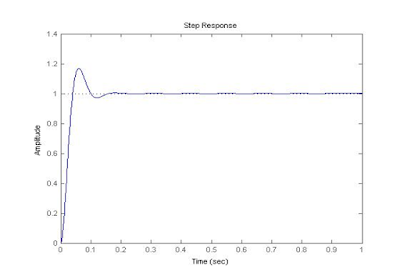## Welcome to the World of Modelling and Simulation

### What is Modelling?

This blog is all about system dynamics modelling and simulation applied in the engineering field, especially mechanical, electrical, and ...### A PID Controller Design Method for DC Motor Speed Control

Control systems analysis and design focuses on three primary objectives:
•        producing the desired transient response
•        achieving stability
Controller: Additional component or device that equalizes or compensates for the performance deficiency is called compensator or controller.Plant: A system to be controlled.
Controller: Provides the excitation for the plant; Designed to control the overall system behavior.
There are several techniques available to the control systems engineer to design a suitable controller.
One of controller widely used is the proportional plus integral plus derivative (PID) controller, which has a transfer function:Kp = Proportional gain
KI = Integral gain
Kd = Derivative gain

The signal (u) just past the controller is now equal to the proportional gain (Kp) times the magnitude of the error plus the integral gain (Ki) times the integral of the error plus the derivative gain (Kd) times the derivative of the error.MATLAB Program:

Design requirements

Settling time less than 1 seconds
Overshoot less than 5%

Open-loop transfer function of the DC Motor:

Proportional Control:

j=3.2284e-6;
b=3.5077e-6;
k=0.0274;
r=4;
l=2.75e-6;
num=k;
kp =1.7;
den=[(j*l) ((j*r)+(l*b)) ((b*r)+k^2)  0];
sys=tf(num,den);
feedbk=feedback(kp*sys,1);
step(feedbk,0:.001:1)

Response Curve:Proportional+Integral Control:

J=0.01;
b=0.1;
K=0.01;
R=1;
L=0.5;
num=K;
den=[(J*L) ((J*R)+(L*b)) ((b*R)+K^2)];
open_sys= tf(num,den);
subplot(2,1,1),step (open_sys,0:0.1:3);
step (open_sys,0:0.01:3);
title('Step Response for the Open Loop System');
kp = 100;
ki = 200;
kd = 0 ;
controller=tf([kd kp ki],[1 0]);
sys=feedback(controller*open_sys,1);
subplot(2,1,2),step (sys,0:0.01:3);
title('Closed loop Step:ki=100 kp=200');

Response Curve:#Blog #Blogger #PID #PIDcontroller #DCmotor #ResponseCurve